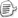1、如何获得一个封闭构造类型（closed constructed type）的Type对象？

class TestType<T>
class TestType<T, U>

//C#
Type t1 = typeof(TestType<int>);
'VB
Dim t2 As Type = GetType(TestType(Of String))

2、如何获取一个泛型类型（generic type）的Type对象？

Type t1 = typeof(TestType<>);
Type t2
= typeof(TestType<,>);
Dim t1, t2 As Type
t1
= GetType(TestType(Of ))
t2
= GetType(TestType(Of ,))

3、如何从构造类型的Type对象生成泛型类型的Type对象？
Type类的新增方法可以做到。
//C#
Type ct = typeof(List<int>);

//Get generic type definition
Type gt = ct.GetGenericTypeDefinition();

4、如何获取类型参数的Type对象？

'VB
Dim t As Type = GetType(List(Of Integer))

'Get the generic arguments, an array
Dim typeArgs As Type() = t.GetGenericArguments()
'Get the first argument: Integer in this case
Dim tArg0 As Type = typeArgs(0)

5、从泛型类型Type对象生成构造类型的Type对象。

//C#
Type ct = typeof(List<int>);
Type gt
= ct.GetGenericTypeDefinition();

//Make another constructed type
//The List<string> in this case
Type ct2 = gt.MakeGenericType(typeof(string));

6、如何取一个开放构造类型（open constructed type）的Type对象？

'The generic type of List(Of T)
Dim tlist As Type = GetType(List(Of ))

'Get the "T" of List(Of T)
Dim typeParam As Type = tlist.GetGenericArguments()(0)

'the generic type of IEnumerable(Of T)
Dim tienum As Type = GetType(IEnumerable(Of ))

'make the open constructed type
Dim tienumOpen As Type = tienum.MakeGenericType(typeParam)

'只有用这种方法获得开放构造类型
'

'

Dim c As ConstructorInfo = _
tlist.GetConstructor(
New Type() {tienumOpen})posted on 2006-06-02 08:48  装配脑袋  阅读(8395)  评论(13编辑  收藏  举报